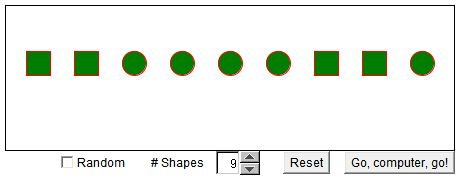# Squares and Circles

In the game of Squares and Circles, the players (you and your computer) start with a sequence of shapes: some circles and some squares. On each move a player selects two shapes. These two are replaced with a single one according to the following rule:

Identical shapes are replaced with a square. Different shapes are replaced with a circle.

At the end of the game, when only one shape remains, you are a winner if the remaining shape is a circle. Otherwise, your computer wins.

If the Random box is checked, computer on Reset will randomly select the length of the sequence (from 3 to 20). When the box is unchecked, you may manually set the number of shapes.

### If you are reading this, your browser is not set to run Java applets. Try IE11 or Safari and declare the site https://www.cut-the-knot.org as trusted in the Java setup.What if applet does not run?

Explanation## References

1. D. Fomin, S. Genkin, I. Itenberg, Mathematical Circles (Russian Experience), AMS, 1996## Explanation

The parity of the number of circles is invariant under any move. Indeed, let, before a move, the number of circles be C and the number of squares S. We have to consider three possibilities:

 Selected After this move circles squares circle,circle C-2 S+1 square,square C S-1 circle,square C S-1

Therefore, if at the outset the number of circles was odd, you win regardless of the sequence of moves. Otherwise, your computer has the upper hand.

(In a little different guise the problem appears elsewhere.)